Home JAQForum Ver 20.06 Log In or Join Active Topics Local Time 01:50 14 Apr 2021 Privacy Policy Jump to Select Sites home page Forum home page Windmills Solar EV's Electronics Microcontrollers PCB Manufacture Other Stuff Site News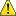Notice. New forum software under development. It's going to miss a few functions and look a bit ugly for a while, but I'm working on it full time now as the old forum was too unstable. Couple days, all good. If you notice any issues, please contact me.

Forum Index : Microcontroller and PC projects : CMM2: V5.07.00b12 - Various fixes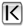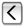Page 5 of 5
Author Message
matherp
Guru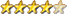Joined: 11/12/2012
Location: United Kingdom
Posts: 4738
 Posted: 08:29am 08 Apr 2021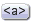Copy link to clipboard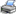Print this post

V5.07.00b26 now available

http://geoffg.net/Downloads/Maximite/CMM2_Beta.zip

GUI BITMAP command restored as per MM2/ArmmiteF4

Mixtel90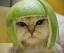Senior MemberJoined: 05/10/2019
Location: United Kingdom
Posts: 123
 Posted: 10:46am 08 Apr 2021Copy link to clipboardPrint this post

ooh! Thanks Peter. I liked that one on the MM2. :)
-- Mick

Zilog Inside! nascom.info for Nascom & Gemini

epsilonSenior MemberJoined: 30/07/2020
Location: Belgium
Posts: 170
 Posted: 08:44am 09 Apr 2021Copy link to clipboardPrint this post

Is this the best place to flag documentation errors?

In the User Guide, the 'pages available in the various modes' next to the mode command has some errors:

MODE 3,16: 0-25 (doc says 0-26)
MODE 3,12: 0-24 (doc says 0-25)
MODE 3,8: 0-53 (doc says 0-54)

MODE 5,16: 0-30 (doc says 0-31)
MODE 5,12: 0-29 (doc says 0-30)
MODE 5,8: 0-60 (doc says 0-61)

MODE 6,16: 0-26 (doc says 0-27)
MODE 6,12: 0-25 (doc says 0-26)
MODE 6,8: 0-53 (doc says 0-54)

MODE 7,16: 0-19 (doc says 0-21)
MODE 7,12: 0-18 (doc says 0-20)
MODE 7,8: 0-41 (doc says 0-42)

(MODEs 11 and 12 I couldn't verify)

MODE 13,16: 0-11 (doc says 0-12)
MODE 13,12: 0-10 (doc says 0-11)
MODE 13,8: 0-26 (doc says 0-27)

MODE 14 is not documented.
Epsilon CMM2 projects

matherp
GuruJoined: 11/12/2012
Location: United Kingdom
Posts: 4738
 Posted: 05:52pm 11 Apr 2021Copy link to clipboardPrint this post

V5.07.00b27 now available

http://geoffg.net/Downloads/Maximite/CMM2_Beta.zip

Improves flac and mp3 playback when using image save

The CMM2 now supports the calculation of pitch, roll and yaw angles from accelerometer and magnetometer inputs.

For  information on this technology see https://github.com/kriswiner/MPU-6050/wiki/Affordable-9-DoF-Sensor-Fusion

The MATH SENSORFUSION command supports both the MADGWICK and MAHONY fusion algorithms. The format of the command  is:
MATH SENSORFUSION type ax, ay, az, gx, gy, gz, mx, my, mz,  pitch, roll, yaw [,p1] [,p2]
Type can be MAHONY or MADGWICK
Ax, ay, and az are the accelerations in the three directions and should be specified in units of standard gravitational acceleration.
Gx, gy, and gz are the instantaneous values of  rotational speed which should be specified in radians per second.
Mx, my, and mz are the magnetic fields in the three directions and should be specified in nano-Tesla (nT)

Care must be taken to ensure that the x, y and z components are consistent between the three inputs. So , for example, using the MPU-9250 the correct input will be ax, ay,az, gx, gy, gz, my, mx, -mz based on the reading from the sensor.

Pitch, roll and yaw should be floating point variables and will contain the outputs from the sensor fusion.

The MATH SENSORFUSION routine will automatically measure the time between consecutive calls and will use this in its internal calculations.

The Madwick algorithm takes an optional parameter p1. This is used as beta in the calculation. It defaults to 0.5 if not specified

The Mahony algorithm takes two optional parameters p1, and p2. These are used as Kp and Ki in the calculation. If not specified these default to 10.0 and 0.0 respectively.

A fully worked example of using the code is given below for the GY-91 MCU9250 module
Connect Vin to 5V, GND to GND, SDA to pin 3, SCL to PIN 5 and SDO to 3.3V (selects the alternative I2C address.

The code contains lots of unnecessary optional features for self test and calibration but the required routines are really just the main program loop, the chip initialisation, and the chip read routines.

Link the roll, pitch and yaw outputs to the CMM2 3D routines to control and visualise a 3D object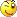'
'  Micromite AHRS using MPU9250
'  Version 2: Sensor fusion converted to MATH SENSORFUSION command
'  Original source code from kriswiner (https://github.com/kriswiner/MPU-9250)
'  and Sebastion Madgwick's Open source IMU and AHRS algorithms
'
'
'   MPU9250 connections
'   GND
'   VCC - 3.3V
'   SCL to I2C clock
'   SDA to I2C data
'   NCS to 3.3V (needed to force I2C)
'   INT to intPin
'   SD0 to GND or 3.3V (see address below)
'
const  screenIO=1 'set to 1 to do fast output on screen, otherwise 0

const MPU9250_ADDRESS =&H69  ' Device address when ADO = 1, must use if RTC also connected to I2C
'const MPU9250_ADDRESS =&H68  ' Device address when ADO = 0
'
'Magnetometer Registers
const AK8963_ADDRESS   =&H0C
const WHO_AM_I_AK8963  =&H00 ' should return =&H48
const AK8963_ST1       =&H02  ' data ready status bit 0
const AK8963_XOUT_L    =&H03  ' data
const AK8963_CNTL      =&H0A  ' Power down (0000), single-measurement (0001), self-test (1000) and Fuse ROM (1111) modes on bits 3:0
const AK8963_ASAX      =&H10  ' Fuse ROM x-axis sensitivity adjustment value
' Gyro/accelerometer registers
const SELF_TEST_X_GYRO =&H00
const SELF_TEST_Y_GYRO =&H01
const SELF_TEST_Z_GYRO =&H02
const SELF_TEST_X_ACCEL =&H0D
const SELF_TEST_Y_ACCEL =&H0E
const SELF_TEST_Z_ACCEL =&H0F
const XG_OFFSET_H      =&H13  ' User-defined trim values for gyroscope
const XG_OFFSET_L      =&H14
const YG_OFFSET_H      =&H15
const YG_OFFSET_L      =&H16
const ZG_OFFSET_H      =&H17
const ZG_OFFSET_L      =&H18
const SMPLRT_DIV       =&H19
const CONFIG           =&H1A
const GYRO_CONFIG      =&H1B
const ACCEL_CONFIG     =&H1C
const ACCEL_CONFIG2    =&H1D
const FIFO_EN          =&H23
const I2C_MST_CTRL     =&H24
const INT_PIN_CFG      =&H37
const INT_ENABLE       =&H38
const INT_STATUS       =&H3A
const ACCEL_XOUT_H     =&H3B
const TEMP_OUT_H       =&H41
const GYRO_XOUT_H      =&H43
const USER_CTRL        =&H6A  ' Bit 7 enable DMP, bit 3 reset DMP
const PWR_MGMT_1       =&H6B ' Device defaults to the SLEEP mode
const PWR_MGMT_2       =&H6C
const FIFO_COUNTH      =&H72
const FIFO_R_W         =&H74
const WHO_AM_I_MPU9250 =&H75 ' Should return =&H71
const XA_OFFSET_H      =&H77
const XA_OFFSET_L      =&H78
const YA_OFFSET_H      =&H7A
const YA_OFFSET_L      =&H7B
const ZA_OFFSET_H      =&H7D
const ZA_OFFSET_L      =&H7E
const   AFS_2G = 0
const   AFS_4G =1
const   AFS_8G =2
const   AFS_16G =3
const   GFS_250DPS = 0
const   GFS_500DPS =1
const   GFS_1000DPS =2
const   GFS_2000DPS =3
const   MFS_14BITS = 0'  0.6 uT per LSB
const   MFS_16BITS =1' 0.15 uT0.6 per LSB
const outputFreq=50.0   ' output frequency in iterations
const  declination=-0.63 ' local magnetic declination in degrees
'
' Global Variable definitions
'
DIM INTEGER Mmode = &H06       ' 1 for single shot, 2 for 8 Hz, 6 for 100 Hz continuous magnetometer data read
DIM INTEGER Gscale = GFS_250DPS
DIM INTEGER Ascale = AFS_4G
DIM INTEGER Mscale = MFS_16BITS ' Choose either 14-bit or 16-bit magnetometer resolution
DIM FLOAT MagCalibration(2), gRes, mRes, aRes
dim float ax, ay, az, gx, gy, gz, mx, my, mz, SelfTest(5), mmult(2)
dim float mxmax=-1000,mxmin=1000,mymax=-1000,mymin=1000,mzmax=-1000,mzmin=1000
dim float pitch,yaw,roll,heading
dim float Kp=10,Ki=0
dim float beta = 0.5 'derived from testing
dim integer gdata(13),mData(6),temp%
DIM INTEGER lastrun
'
' Calibration parameters for gyro and accelerometer
dim float gyro_Bias(2)=(40,15,100), accel_Bias(2)=(340,55,-390)
'
' calibration parameters for magnetometer derived from running AK8963calibrate
DIM float magbias(2)=(-20.205,7.035,18.165), magscale(2)=(1,1,1)
'
'  Setup
'
i2c open 400,1000
pause 100
writeByte(MPU9250_ADDRESS,PWR_MGMT_1,&H80)
pause 500
initMPU9250
temp%=readByte(MPU9250_ADDRESS,WHO_AM_I_MPU9250)
if temp%<>&H71 then
Print "MPU9250 not found"
end
endif
temp%=readByte(AK8963_ADDRESS,WHO_AM_I_AK8963)
if temp%<>&H48 then
Print "AK8963 not found"
end
endif
print "Internal temperature is ",readTempData()
if screenIO then cls
initAK8963(MagCalibration())
getAres
getGres
getMres
if screenIO then readMPU9250(0)
' Uncomment this section to run  the self test algorithm of the accelerometer and gyro
' Ensure the module is on a level surface and is completely still
' MPU9250SelfTest(selftest())
' print "Errors in %"
' print "    Gyro    Accel"
' Print "X ",str\$(selftest(3),3,3," "),STR\$(selftest(0),4,3," ")
' Print "Y ",str\$(selftest(4),3,3," "),STR\$(selftest(1),4,3," ")
' Print "Z ",str\$(selftest(5),3,3," "),STR\$(selftest(2),4,3," ")
' end
'
' Uncomment this section to derive calibration parameters for gyro and accelerometer
' Ensure the module is on a level surface and is completely still
' note the parameters and set them to load into the arrays gyroBias and accelBias above
' initMPU9250
' calibrateMPU9250(gyroBias(), accelBias())
' print "    Gyro    Accel"
' Print "X ",str\$(gyroBias(0),3,3," "),STR\$(accelbias(0),4,3," ")
' Print "Y ",str\$(gyroBias(1),3,3," "),STR\$(accelbias(1),4,3," ")
' Print "Z ",str\$(gyroBias(2),3,3," "),STR\$(accelbias(2),4,3," ")
' end
'

initMPU9250
loadcalibrations
'Print "AK8963 mag calibration X=",MagCalibration(0),"  Y=",MagCalibration(1),"  Z=",MagCalibration(2)
mmult(0)=mRes*magCalibration(0)/magscale(0)
mmult(1)=mRes*magCalibration(1)/magscale(1)
mmult(2)=mRes*magCalibration(2)/magscale(2)
temp%=0
'
' Main program
'
timer=0
do

readMPU9250(0)

'  SensorFusion Madgwick ax, ay, az, gx, gy, gz, mx, my, mz, pitch, roll, yaw', beta
math  SensorFusion Mahony ax, ay, az, gx, gy, gz, mx, my, mz,  pitch, roll, yaw', Kp, Ki

heading   = ((720.0-yaw*180/PI)- declination)
heading = heading mod 360.0
if (temp% mod outputFreq) = 0 then
Print @(0,4*mm.info(fontheight))"Heading = "+str\$(heading,3,1," ")
endif
temp%=temp%+1
if screenIO then text 0,100,"pitch="+str\$(pitch*180/PI,3,2," ")+"  roll="+str\$(roll*180/PI,3,2," ")+"  yaw="+str\$(yaw*180/PI,3,2," ")

loop
end
'
sub readMPU9250(offset%)
local integer i
local float j
do
i=readByte(MPU9250_ADDRESS, INT_STATUS) and readByte(AK8963_ADDRESS, AK8963_ST1) and 1
loop until i=1

readBytes(MPU9250_ADDRESS, ACCEL_XOUT_H, 14, gData()) 'read in the gyro and accelerometer data
readBytes(AK8963_ADDRESS, AK8963_XOUT_L, 7, mData())' Read the six raw data and ST2 registers sequentially into data array

if((mData(6) AND &H08)=0) THEN ' Check if magnetic sensor overflow set, if not then report data
i=(mData(1) << 8) OR mData(0):IF i AND &H8000 then i=i OR &HFFFFFFFFFFFF0000
my = i
my = my * mmult(0) + magbias(0)' Turn the MSB and LSB into a signed 16-bit value
i=(mData(3) << 8) OR mData(2):IF i AND &H8000 then i=i OR &HFFFFFFFFFFFF0000
mx = i
mx=mx * mmult(1) + magbias(1) ' Data stored as little Endian
i=(mData(5) << 8) OR mData(4):IF i AND &H8000 then i=i OR &HFFFFFFFFFFFF0000
mz = i
mz=-(mz * mmult(2) + magbias(2))
'    if mx>mxmax then mxmax=mx
'    if my>mymax then mymax=my
'    if mz>mzmax then mzmax=mz
'    if mx<mxmin then mxmin=mx
'    if my<mymin then mymin=my
'    if mz<mzmin then mzmin=mz
endif
' Now we'll calculate the acceleration value into actual g's
' this depends on scale being set
i=(gData(0) << 8) OR gData(1):IF i AND &H8000 then i=i OR &HFFFFFFFFFFFF0000
ax = i
ax=ax*aRes ' Turn the MSB and LSB into a signed 16-bit value
i=(gData(2) << 8) OR gData(3):IF i AND &H8000 then i=i OR &HFFFFFFFFFFFF0000
ay = i
ay=ay*aRes
i=(gData(4) << 8) OR gData(5):IF i AND &H8000 then i=i OR &HFFFFFFFFFFFF0000
az = i
az=az*aRes

' Calculate the gyro value into actual radians per second
i=(gData(8) << 8) OR gData(9):IF i AND &H8000 then i=i OR &HFFFFFFFFFFFF0000
j=i
gx = RAD(j* gRes) 'Turn the MSB and LSB into a signed 16-bit value
i=(gData(10) << 8) OR gData(11):IF i AND &H8000 then i=i OR &HFFFFFFFFFFFF0000
j=i
gy = RAD(j* gRes)
i=(gData(12) << 8) OR gData(13):IF i AND &H8000 then i=i OR &HFFFFFFFFFFFF0000
j=i
gz = RAD(j* gRes)
if screenIO then text 0,0+offset%,"Acc X="+str\$(ax,3,2," ")+"  Y="+str\$(ay,3,2," ")+"  Z="+str\$(az,3,2," ")
if screenIO then text 0,13+offset%,"Rot X="+str\$(gx,3,2," ")+"  Y="+str\$(gy,3,2," ")+"  Z="+str\$(gz,3,2," ")
if screenIO then text 0,26+offset%,"Mag X="+str\$(mx,3,2," ")+"  Y="+str\$(my,3,2," ")+"  Z="+str\$(mz,3,2," ")
'    if screenIO then text 0,39+offset%,"Max X="+str\$(mxmax,3,2," ")+"  Y="+str\$(mymax,3,2," ")+"  Z="+str\$(mzmax,3,2," ")
'    if screenIO then text 0,52+offset%,"Min X="+str\$(mxmin,3,2," ")+"  Y="+str\$(mymin,3,2," ")+"  Z="+str\$(mzmin,3,2," ")

end sub
'
sub initMPU9250
LOCAL INTEGER c
' wake up device
writeByte(MPU9250_ADDRESS, PWR_MGMT_1, &H80) ' Reset
pause 100 ' Wait for all registers to reset
writeByte(MPU9250_ADDRESS, PWR_MGMT_1, &H00) ' Clear sleep mode bit (6), enable all sensors
pause 100 ' Wait for all registers to reset
' get stable time source
writeByte(MPU9250_ADDRESS, PWR_MGMT_1, &H01)  ' Auto select clock source to be PLL gyroscope reference if ready else
pause 200
' Configure Gyro and Thermometer
' Disable FSYNC and set thermometer and gyro bandwidth to 41 and 42 Hz, respectively
' minimum delay time for this setting is 5.9 ms, which means sensor fusion update rates cannot
' be higher than 1 / 0.0059 = 170 Hz
' DLPF_CFG = bits 2:0 = 011 this limits the sample rate to 1000 Hz for both
' With the MPU9250, it is possible to get gyro sample rates of 32 kHz (!), 8 kHz, or 1 kHz
writeByte(MPU9250_ADDRESS, CONFIG, &H03)
' Set sample rate = gyroscope output rate/(1 + SMPLRT_DIV)
writeByte(MPU9250_ADDRESS, SMPLRT_DIV, &H04)  ' Use a 200 Hz rate a rate consistent with the filter update rate
' Set gyroscope full scale range
' Range selects FS_SEL and AFS_SEL are 0 - 3, so 2-bit values are left-shifted into positions 4:3
c = readByte(MPU9250_ADDRESS, GYRO_CONFIG)
c=c AND &B11100100
c = c OR (Gscale << 3) ' Set full scale range for the gyro
writeByte(MPU9250_ADDRESS, GYRO_CONFIG, c ) ' Write new GYRO_CONFIG value to register
' Set accelerometer full-scale range configuration
c = readByte(MPU9250_ADDRESS, ACCEL_CONFIG)
c=c AND &B11100111
c =  c OR (Ascale << 3) ' Set full scale range for the accelerometer
writeByte(MPU9250_ADDRESS, ACCEL_CONFIG, c) ' Write new ACCEL_CONFIG register value
' Set accelerometer sample rate configuration
' It is possible to get a 4 kHz sample rate from the accelerometer by choosing 1 for
' accel_fchoice_b bit (3) in this case the bandwidth is 1.13 kHz
c = readByte(MPU9250_ADDRESS, ACCEL_CONFIG2)
c = c AND &B11110000
c = c OR &H03  ' Set accelerometer rate to 1 kHz and bandwidth to 41 Hz
writeByte(MPU9250_ADDRESS, ACCEL_CONFIG2, c) ' Write new ACCEL_CONFIG2 register value
' The accelerometer, gyro, and thermometer are set to 1 kHz sample rates,
' but all these rates are further reduced by a factor of 5 to 200 Hz because of the SMPLRT_DIV setting
' Configure Interrupts and Bypass Enable
' Set interrupt pin active high, push-pull, hold interrupt pin level HIGH until interrupt cleared,
' clear on read of INT_STATUS, and enable I2C_BYPASS_EN so additional chips
' can join the I2C bus and all can be controlled by the Arduino as master
writeByte(MPU9250_ADDRESS, INT_PIN_CFG, &H32)
writeByte(MPU9250_ADDRESS, INT_ENABLE, &H01)  ' Enable data ready (bit 0) interrupt
writeByte(MPU9250_ADDRESS, USER_CTRL, &H05)
pause 100
end sub

sub initAK8963(destination() as float)
' First extract the factory calibration for each magnetometer axis
local integer rawData(2); ' x/y/z gyro calibration data stored here
writeByte(AK8963_ADDRESS, AK8963_CNTL, &H00); ' Power down magnetometer
Pause 10
writeByte(AK8963_ADDRESS, AK8963_CNTL, &H0F); ' Enter Fuse ROM access mode
readBytes(AK8963_ADDRESS, AK8963_ASAX, 3, rawData());  ' Read the x-, y-, and z-axis calibration values
destination(0) =  (rawData(0) - 128)/256 + 1   ' Return x-axis sensitivity adjustment values, etc.
destination(1) =  (rawData(1) - 128)/256 + 1
destination(2) =  (rawData(2) - 128)/256 + 1
writeByte(AK8963_ADDRESS, AK8963_CNTL, &H00); ' Power down magnetometer
Pause 10
' Configure the magnetometer for continuous read and highest resolution
' set Mscale bit 4 to 1 (0) to enable 16 (14) bit resolution in CNTL register,
' and enable continuous mode data acquisition Mmode (bits [3:0]), 0010 for 8 Hz and 0110 for 100 Hz sample rates
writeByte(AK8963_ADDRESS, AK8963_CNTL, (Mscale << 4) OR Mmode) 'Set magnetometer data resolution and sample ODR
Pause 10
end sub

sub writeByte(address%,reg%,value%)
i2c write address%,0,2,reg%,value%
end sub
'
function readByte(address%,reg%) as integer
i2c write address%,1,1,reg%
i2c read address%,0,1,readByte
end function
'
sub readBytes(address%,reg%,n%,a%()) as integer
i2c write address%,1,1,reg%
i2c read address%,0,n%,a%()
end sub
'
sub getMres
select case Mscale
' Possible magnetometer scales (and their register bit settings) are:
' 14 bit resolution (0) and 16 bit resolution (1)
case MFS_14BITS
mRes = 0.6 ' Proper scale to return milliGauss
case MFS_16BITS
mRes = 0.15 ' Proper scale to return milliGauss
end select
end sub
'
sub getGres
select case Gscale
' Possible gyro scales (and their register bit settings) are:
'250 DPS (00), 500 DPS (01), 1000 DPS (10), and 2000 DPS  (11).
// Here's a bit of an algorith to calculate DPS/(ADC tick) based on that 2-bit value:
case GFS_250DPS
gRes = 250.0/32768.0
case GFS_500DPS
gRes = 500.0/32768.0
case GFS_1000DPS
gRes = 1000.0/32768.0
case GFS_2000DPS
gRes = 2000.0/32768.0
end select
end sub
'
sub getAres
select case Ascale
' Possible accelerometer scales (and their register bit settings) are:
' 2 Gs (00), 4 Gs (01), 8 Gs (10), and 16 Gs  (11).
// Here's a bit of an algorith to calculate DPS/(ADC tick) based on that 2-bit value:
case AFS_2G
aRes = 2.0/32768.0
case AFS_4G
aRes = 4.0/32768.0
case AFS_8G
aRes = 8.0/32768.0
case AFS_16G:
aRes = 16.0/32768.0
end select
end sub
'
function readTempData() as float
local integer rawData(1)  ' x/y/z gyro register data stored here
readBytes(MPU9250_ADDRESS, TEMP_OUT_H, 2, rawData()) ' Read the two raw data registers sequentially into data array
readTempData=sint16((rawData(0) << 8) OR rawData(1)) / 333.87 + 21.0 '
end function
'
FUNCTION sint16(x as integer) as float ' convert to signed 16 bit number
local a%=x
IF a% AND &H8000 then a%=a% OR &HFFFFFFFFFFFF0000
sint16 = a%
END FUNCTION
'
FUNCTION iint16(x as integer) as integer ' convert to signed 16 bit number
local a%=x
IF a% AND &H8000 then a%=a% OR &HFFFFFFFFFFFF0000
iint16 = a%
END FUNCTION
'
FUNCTION iint15(x as integer) as integer ' convert to signed 16 bit number
local a%=x
IF a% AND &H4000 then a%=a% OR &HFFFFFFFFFFFF8000
iint15 = a%
END FUNCTION
'
sub MPU9250SelfTest(destination() as float) ' Should return percent deviation from factory trim values, +/- 14 or less deviation is a pass
local integer rawData(5) = (0, 0, 0, 0, 0, 0)
local integer selfTest(5)
local integer gAvg(2)=(0,0,0), aAvg(2)=(0,0,0), aSTAvg(2)=(0,0,0), gSTAvg(2)=(0,0,0)
local float factoryTrim(5)
local integer FS = 0,ii
writeByte(MPU9250_ADDRESS, SMPLRT_DIV, &H00)    ' Set gyro sample rate to 1 kHz
writeByte(MPU9250_ADDRESS, CONFIG, &H02)        ' Set gyro sample rate to 1 kHz and DLPF to 92 Hz
writeByte(MPU9250_ADDRESS, GYRO_CONFIG, (1<<FS))  ' Set full scale range for the gyro to 250 dps
writeByte(MPU9250_ADDRESS, ACCEL_CONFIG2, &H02) ' Set accelerometer rate to 1 kHz and bandwidth to 92 Hz
writeByte(MPU9250_ADDRESS, ACCEL_CONFIG, (1<<FS)) ' Set full scale range for the accelerometer to 2 g
'
for ii = 0 to 199  ' get average current values of gyro and acclerometer
readBytes(MPU9250_ADDRESS, ACCEL_XOUT_H, 6, rawData())        ' Read the six raw data registers into data array
aAvg(0) = aAvg(0) + iint16((rawData(0) << 8) OR rawData(1))   ' Turn the MSB and LSB into a signed 16-bit value
aAvg(1) = aAvg(1) + iint16((rawData(2) << 8) OR rawData(3))
aAvg(2) = aAvg(2) + iint16((rawData(4) << 8) OR rawData(5))
readBytes(MPU9250_ADDRESS, GYRO_XOUT_H, 6, rawData())       ' Read the six raw data registers sequentially into data array
gAvg(0) = gAvg(0) + iint16((rawData(0) << 8) OR rawData(1))   ' Turn the MSB and LSB into a signed 16-bit value
gAvg(1) = gAvg(1) + iint16((rawData(2) << 8) OR rawData(3))
gAvg(2) = gAvg(2) + iint16((rawData(4) << 8) OR rawData(5))
next ii
'
for ii =0 to 2 ' Get average of 200 values and store as average current readings
aAvg(ii) = aAvg(ii)/ 200
gAvg(ii) = gAvg(ii)/ 200
next ii
' Configure the accelerometer for self-test
writeByte(MPU9250_ADDRESS, ACCEL_CONFIG, &HE0) ' Enable self test on all three axes and set accelerometer range to +/- 2 g
writeByte(MPU9250_ADDRESS, GYRO_CONFIG,  &HE0) ' Enable self test on all three axes and set gyro range to +/- 250 degrees/s
pause 25  ' Delay a while to let the device stabilize
for ii = 0 to 199   ' get average self-test values of gyro and acclerometer
readBytes(MPU9250_ADDRESS, ACCEL_XOUT_H, 6, rawData())  ' Read the six raw data registers into data array
aSTAvg(0) = aSTAvg(0) + iint16((rawData(0) << 8) OR rawData(1))   ' Turn the MSB and LSB into a signed 16-bit value
aSTAvg(1) = aSTAvg(1) + iint16((rawData(2) << 8) OR rawData(3))
aSTAvg(2) = aSTAvg(2) + iint16((rawData(4) << 8) OR rawData(5))
readBytes(MPU9250_ADDRESS, GYRO_XOUT_H, 6, rawData())  ' Read the six raw data registers sequentially into data array
gSTAvg(0) = gSTAvg(0) + iint16((rawData(0) << 8) OR rawData(1))  ' Turn the MSB and LSB into a signed 16-bit value
gSTAvg(1) = gSTAvg(1) + iint16((rawData(2) << 8) OR rawData(3))
gSTAvg(2) = gSTAvg(2) + iint16((rawData(4) << 8) OR rawData(5))
next ii
'
for ii =0 to 2 ' Get average of 200 values and store as average current readings
aSTAvg(ii) =aSTAvg(ii)/200
gSTAvg(ii) =gSTAvg(ii)/200
next ii
'
' Configure the gyro and accelerometer for normal operation
writeByte(MPU9250_ADDRESS, ACCEL_CONFIG, &H00)
writeByte(MPU9250_ADDRESS, GYRO_CONFIG,  &H00)
pause 25  ' Delay a while to let the device stabilize
'
' Retrieve accelerometer and gyro factory Self-Test Code from USR_Reg
selfTest(0) = (readByte(MPU9250_ADDRESS, SELF_TEST_X_ACCEL)) ' X-axis accel self-test results
selfTest(1) = (readByte(MPU9250_ADDRESS, SELF_TEST_Y_ACCEL)) ' Y-axis accel self-test results
selfTest(2) = (readByte(MPU9250_ADDRESS, SELF_TEST_Z_ACCEL)) ' Z-axis accel self-test results
selfTest(3) = (readByte(MPU9250_ADDRESS, SELF_TEST_X_GYRO) ) ' X-axis gyro self-test results
selfTest(4) = (readByte(MPU9250_ADDRESS, SELF_TEST_Y_GYRO) ) ' Y-axis gyro self-test results
selfTest(5) = (readByte(MPU9250_ADDRESS, SELF_TEST_Z_GYRO) ) ' Z-axis gyro self-test results
'
' Retrieve factory self-test value from self-test code reads
factoryTrim(0) = (2620/(1<<FS))*(( 1.01 ^ (selfTest(0) - 1.0) )) ' FT(Xa) factory trim calculation
factoryTrim(1) = (2620/(1<<FS))*(( 1.01 ^ (selfTest(1) - 1.0) )) ' FT(Ya) factory trim calculation
factoryTrim(2) = (2620/(1<<FS))*(( 1.01 ^ (selfTest(2) - 1.0) )) ' FT(Za) factory trim calculation
factoryTrim(3) = (2620/(1<<FS))*(( 1.01 ^ (selfTest(3) - 1.0) )) ' FT(Xg) factory trim calculation
factoryTrim(4) = (2620/(1<<FS))*(( 1.01 ^ (selfTest(4) - 1.0) )) ' FT(Yg) factory trim calculation
factoryTrim(5) = (2620/(1<<FS))*(( 1.01 ^ (selfTest(5) - 1.0) )) ' FT(Zg) factory trim calculation
'
' Report results as a ratio of (STR - FT)/FT the change from Factory Trim of the Self-Test Response
' To get percent, must multiply by 100
for ii=0 to 2
destination(ii)   = (aAvg(ii)-aSTAvg(ii)+factoryTrim(ii)) / factoryTrim(ii)*100   ' Report percent differences
destination(ii+3) = (gAvg(ii)-gSTAvg(ii)+factoryTrim(ii+3)) / factoryTrim(ii+3)*100 ' Report percent differences
next ii
end sub
'
sub calibrateMPU9250(dest1() as float, dest2() as float)
LOCAL INTEGER rData(11) ' data array to hold accelerometer and gyro x, y, z, data
LOCAL INTEGER ii, jj, packet_count, fifo_count
LOCAL INTEGER gyro_bias(2)  = (0, 0, 0), accel_bias(2) = (0, 0, 0),accel_temp(2),gyro_temp(2)
LOCAL INTEGER accel_bias_reg(2) = (0, 0, 0)' A place to hold the factory accelerometer trim biases
LOCAL INTEGER mask_bit(2) = (0, 0, 0) ' Define array to hold mask bit for each accelerometer bias axis
LOCAL INTEGER  accelsensitivity = 16384  ' = 16384 LSB/g
'
' reset device
writeByte(MPU9250_ADDRESS, PWR_MGMT_1, &H80) ' Write a one to bit 7 reset bit toggle reset device
pause 100
'
' get stable time source Auto select clock source to be PLL gyroscope reference if ready
' else use the internal oscillator, bits 2:0 = 001
writeByte(MPU9250_ADDRESS, PWR_MGMT_1, &H01)
writeByte(MPU9250_ADDRESS, PWR_MGMT_2, &H00)
pause 200
'
' Configure device for bias calculation
writeByte(MPU9250_ADDRESS, INT_ENABLE, &H00)   ' Disable all interrupts
writeByte(MPU9250_ADDRESS, FIFO_EN, &H00)      ' Disable FIFO
writeByte(MPU9250_ADDRESS, PWR_MGMT_1, &H00)   ' Turn on internal clock source
writeByte(MPU9250_ADDRESS, I2C_MST_CTRL, &H00) ' Disable I2C master
writeByte(MPU9250_ADDRESS, USER_CTRL, &H00)    ' Disable FIFO and I2C master modes
writeByte(MPU9250_ADDRESS, USER_CTRL, &H0C)    ' Reset FIFO and DMP
pause 15
'
' Configure MPU6050 gyro and accelerometer for bias calculation
writeByte(MPU9250_ADDRESS, CONFIG, &H01)      ' Set low-pass filter to 188 Hz
writeByte(MPU9250_ADDRESS, SMPLRT_DIV, &H00)  ' Set sample rate to 1 kHz
writeByte(MPU9250_ADDRESS, GYRO_CONFIG, &H00)  ' Set gyro full-scale to 250 degrees per second, maximum sensitivity
writeByte(MPU9250_ADDRESS, ACCEL_CONFIG, &H00) ' Set accelerometer full-scale to 2 g, maximum sensitivity

' Configure FIFO to capture accelerometer and gyro data for bias calculation
writeByte(MPU9250_ADDRESS, USER_CTRL, &H40)   ' Enable FIFO
writeByte(MPU9250_ADDRESS, FIFO_EN, &H78)     ' Enable gyro and accelerometer sensors for FIFO  (max size 512 bytes in MPU-9150)
pause 40 ' accumulate 40 samples in 40 milliseconds = 480 bytes
'
' At end of sample accumulation, turn off FIFO sensor read
writeByte(MPU9250_ADDRESS, FIFO_EN, &H00)        ' Disable gyro and accelerometer sensors for FIFO
readBytes(MPU9250_ADDRESS, FIFO_COUNTH, 2, rData()) ' read FIFO sample count
fifo_count = ((rData(0) << 8) OR rData(1))
packet_count = fifo_count/12' How many sets of full gyro and accelerometer data for averaging
for ii=0 to packet_count-1
for jj=0 to 2
accel_temp(jj)=0
gyro_temp(jj)=0
next jj
readBytes(MPU9250_ADDRESS, FIFO_R_W, 12, rData()) ' read data for averaging
accel_temp(0) =  iint16((rData(0) << 8) OR rData(1)  )   ' Form signed 16-bit integer for each sample in FIFO
accel_temp(1) =  iint16((rData(2) << 8) OR rData(3)  )
accel_temp(2) =  iint16((rData(4) << 8) OR rData(5)  )
gyro_temp(0)  =  iint16((rData(6) << 8) OR rData(7)  )
gyro_temp(1)  =  iint16((rData(8) << 8) OR rData(9)  )
gyro_temp(2)  =  iint16((rData(10) << 8) OR rData(11))

accel_bias(0) = accel_bias(0)+ accel_temp(0) ' Sum individual signed 16-bit biases to get accumulated signed 32-bit biases
accel_bias(1) = accel_bias(1)+ accel_temp(1)
accel_bias(2) = accel_bias(2)+ accel_temp(2)
gyro_bias(0) = gyro_bias(0)+ gyro_temp(0)
gyro_bias(1) = gyro_bias(1)+ gyro_temp(1)
gyro_bias(2) = gyro_bias(2)+ gyro_temp(2)
next ii
accel_bias(0) = accel_bias(0)/ packet_count ' Normalize sums to get average count biases
accel_bias(1) = accel_bias(1)/ packet_count
accel_bias(2) = accel_bias(2)/ packet_count
gyro_bias(0) = gyro_bias(0)/ packet_count
gyro_bias(1) = gyro_bias(1)/ packet_count
gyro_bias(2) = gyro_bias(2)/ packet_count
if(accel_bias(2) > 0) THEN
accel_bias(2) = accel_bias(2) - accelsensitivity  ' Remove gravity from the z-axis accelerometer bias calculation
else
accel_bias(2) = accel_bias(2) + accelsensitivity
endif
gyro_bias(0)=gyro_bias(0)/-4
gyro_bias(1)=gyro_bias(1)/-4
gyro_bias(2)=gyro_bias(2)/-4
' Construct the gyro biases for push to the hardware gyro bias registers, which are reset to zero upon device startup
rData(0) = (gyro_bias(0)>>8) AND &HFF ' Divide by 4 to get 32.9 LSB per deg/s to conform to expected bias input format
rData(1) = gyro_bias(0)      AND &HFF ' Biases are additive, so change sign on calculated average gyro biases
rData(2) = (gyro_bias(1)>>8) AND &HFF
rData(3) = gyro_bias(1)     AND &HFF
rData(4) = (gyro_bias(2)>>8) AND &HFF
rData(5) = gyro_bias(2)      AND &HFF
'
' Push gyro biases to hardware registers
writeByte(MPU9250_ADDRESS, XG_OFFSET_H, rData(0))
writeByte(MPU9250_ADDRESS, XG_OFFSET_L, rData(1))
writeByte(MPU9250_ADDRESS, YG_OFFSET_H, rData(2))
writeByte(MPU9250_ADDRESS, YG_OFFSET_L, rData(3))
writeByte(MPU9250_ADDRESS, ZG_OFFSET_H, rData(4))
writeByte(MPU9250_ADDRESS, ZG_OFFSET_L, rData(5))
'
' Output scaled gyro biases for display in the main program
dest1(0) = gyro_bias(0)
dest1(1) = gyro_bias(1)
dest1(2) = gyro_bias(2)
'
' Construct the accelerometer biases for push to the hardware accelerometer bias registers. These registers contain
' factory trim values which must be added to the calculated accelerometer biases on boot up these registers will hold
' non-zero values. In addition, bit 0 of the lower byte must be preserved since it is used for temperature
' compensation calculations. Accelerometer bias registers expect bias input as 2048 LSB per g, so that
' the accelerometer biases calculated above must be divided by 8.
'
readBytes(MPU9250_ADDRESS, XA_OFFSET_H, 2, rData()) ' Read factory accelerometer trim values
accel_bias_reg(0) =  iint15((rData(0) << 7) OR ((rData(1) and &HFE)>>1))
if rData(1) and &H1 then mask_bit(0)=&H1
readBytes(MPU9250_ADDRESS, YA_OFFSET_H, 2, rData())
accel_bias_reg(1) =  iint15((rData(0) << 7) OR ((rData(1) and &HFE)>>1))
if rData(1) and &H1 then mask_bit(1)=&H1
readBytes(MPU9250_ADDRESS, ZA_OFFSET_H, 2, rData())
accel_bias_reg(2) =  iint15((rData(0) << 7) OR ((rData(1) and &HFE)>>1))
if rData(1) and &H1 then mask_bit(2)=&H1
'
' Construct total accelerometer bias, including calculated average accelerometer bias from above
accel_bias_reg(0) = accel_bias_reg(0) - (accel_bias(0)\16) ' Subtract calculated averaged accelerometer bias scaled to 2048 LSB/g (16 g full scale)
accel_bias_reg(1) = accel_bias_reg(1) - (accel_bias(1)\16)
accel_bias_reg(2) = accel_bias_reg(2) - (accel_bias(2)\16)
rData(0) = (accel_bias_reg(0) \ 128) AND &HFF
rData(1) = ((accel_bias_reg(0))<<1 )     AND &HFE
rData(1) = rData(1) OR mask_bit(0) ' preserve temperature compensation bit when writing back to accelerometer bias registers
rData(2) = (accel_bias_reg(1) \ 128) AND &HFF
rData(3) = ((accel_bias_reg(1)) <<1)     AND &HFE
rData(3) = rData(3) OR mask_bit(1) ' preserve temperature compensation bit when writing back to accelerometer bias registers
rData(4) = (accel_bias_reg(2) \ 128) AND &HFF
rData(5) = ((accel_bias_reg(2))<<1)      AND &HFE
rData(5) = rData(5) OR mask_bit(2) ' preserve temperature compensation bit when writing back to accelerometer bias registers
'
' Push accelerometer biases to hardware registers
writeByte(MPU9250_ADDRESS, XA_OFFSET_H, rData(0))
writeByte(MPU9250_ADDRESS, XA_OFFSET_L, rData(1))
writeByte(MPU9250_ADDRESS, YA_OFFSET_H, rData(2))
writeByte(MPU9250_ADDRESS, YA_OFFSET_L, rData(3))
writeByte(MPU9250_ADDRESS, ZA_OFFSET_H, rData(4))
writeByte(MPU9250_ADDRESS, ZA_OFFSET_L, rData(5))
' Output scaled accelerometer biases for display in the main program
dest2(0) = accel_bias(0)
dest2(1) = accel_bias(1)
dest2(2) = accel_bias(2)
print "calibration done"
end sub
'
sub loadcalibrations
LOCAL INTEGER mask_bit(2) = (0, 0, 0) ' Define array to hold mask bit for each accelerometer bias axis
LOCAL INTEGER accel_bias_reg(2) = (0, 0, 0)' A place to hold the factory accelerometer trim biases
LOCAL INTEGER rData(11) ' data array to hold accelerometer and gyro x, y, z, data
' Construct the gyro biases for push to the hardware gyro bias registers, which are reset to zero upon device startup
rData(0) = (gyro_bias(0)>>8) AND &HFF ' Divide by 4 to get 32.9 LSB per deg/s to conform to expected bias input format
rData(1) = gyro_bias(0)      AND &HFF ' Biases are additive, so change sign on calculated average gyro biases
rData(2) = (gyro_bias(1)>>8) AND &HFF
rData(3) = gyro_bias(1)     AND &HFF
rData(4) = (gyro_bias(2)>>8) AND &HFF
rData(5) = gyro_bias(2)      AND &HFF
'
' Push gyro biases to hardware registers
writeByte(MPU9250_ADDRESS, XG_OFFSET_H, rData(0))
writeByte(MPU9250_ADDRESS, XG_OFFSET_L, rData(1))
writeByte(MPU9250_ADDRESS, YG_OFFSET_H, rData(2))
writeByte(MPU9250_ADDRESS, YG_OFFSET_L, rData(3))
writeByte(MPU9250_ADDRESS, ZG_OFFSET_H, rData(4))
writeByte(MPU9250_ADDRESS, ZG_OFFSET_L, rData(5))
'
'
readBytes(MPU9250_ADDRESS, XA_OFFSET_H, 2, rData()) ' Read factory accelerometer trim values
accel_bias_reg(0) =  iint15((rData(0) << 7) OR ((rData(1) and &HFE)>>1))
if rData(1) and &H1 then mask_bit(0)=&H1
readBytes(MPU9250_ADDRESS, YA_OFFSET_H, 2, rData())
accel_bias_reg(1) =  iint15((rData(0) << 7) OR ((rData(1) and &HFE)>>1))
if rData(1) and &H1 then mask_bit(1)=&H1
readBytes(MPU9250_ADDRESS, ZA_OFFSET_H, 2, rData())
accel_bias_reg(2) =  iint15((rData(0) << 7) OR ((rData(1) and &HFE)>>1))
if rData(1) and &H1 then mask_bit(2)=&H1
'
' Construct total accelerometer bias, including calculated average accelerometer bias from above
accel_bias_reg(0) = accel_bias_reg(0) - (accel_bias(0)\16) ' Subtract calculated averaged accelerometer bias scaled to 2048 LSB/g (16 g full scale)
accel_bias_reg(1) = accel_bias_reg(1) - (accel_bias(1)\16)
accel_bias_reg(2) = accel_bias_reg(2) - (accel_bias(2)\16)
rData(0) = (accel_bias_reg(0) \ 128) AND &HFF
rData(1) = ((accel_bias_reg(0))<<1 )     AND &HFE
rData(1) = rData(1) OR mask_bit(0) ' preserve temperature compensation bit when writing back to accelerometer bias registers
rData(2) = (accel_bias_reg(1) \ 128) AND &HFF
rData(3) = ((accel_bias_reg(1)) <<1)     AND &HFE
rData(3) = rData(3) OR mask_bit(1) ' preserve temperature compensation bit when writing back to accelerometer bias registers
rData(4) = (accel_bias_reg(2) \ 128) AND &HFF
rData(5) = ((accel_bias_reg(2))<<1)      AND &HFE
rData(5) = rData(5) OR mask_bit(2) ' preserve temperature compensation bit when writing back to accelerometer bias registers
'
' Push accelerometer biases to hardware registers
writeByte(MPU9250_ADDRESS, XA_OFFSET_H, rData(0))
writeByte(MPU9250_ADDRESS, XA_OFFSET_L, rData(1))
writeByte(MPU9250_ADDRESS, YA_OFFSET_H, rData(2))
writeByte(MPU9250_ADDRESS, YA_OFFSET_L, rData(3))
writeByte(MPU9250_ADDRESS, ZA_OFFSET_H, rData(4))
writeByte(MPU9250_ADDRESS, ZA_OFFSET_L, rData(5))
end sub

Edited 2021-04-12 03:57 by matherp

retepsnikrep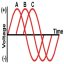Senior MemberJoined: 31/12/2007
Location: United Kingdom
Posts: 121
 Posted: 06:38pm 11 Apr 2021Copy link to clipboardPrint this post

My brain has yawed off to the left and right before exploding with that latest post.
Incredible work..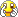Gen1 Honda Insights.

epsilonSenior MemberJoined: 30/07/2020
Location: Belgium
Posts: 170
 Posted: 08:34pm 11 Apr 2021Copy link to clipboardPrint this post

Apologies for messing up the thread, but this thread is probably still the best place to flag issues.

I noticed that the COMMENT directive doesn't work when put inside a .INC:

SUB foo
PRINT "In foo"
#COMMENT START
PRINT "In foo comment"
#COMMENT END
END SUB

In foo
Error in test.inc line 4: Invalid character: #

matherp said  V5.07.00b21 now available

New directive to allow multi-line comments. The commands must be in capitals. Any lines between the two commands are completely ignored and not loaded into memory
#COMMENT START
#COMMENT END

Epsilon CMM2 projects

matherp
GuruJoined: 11/12/2012
Location: United Kingdom
Posts: 4738
 Posted: 09:43pm 11 Apr 2021Copy link to clipboardPrint this post

Quote  I noticed that the COMMENT directive doesn't work when put inside a .INC:

Cut and paste error - I'll fix in the next beta

epsilonSenior MemberJoined: 30/07/2020
Location: Belgium
Posts: 170
 Posted: 06:59am 12 Apr 2021Copy link to clipboardPrint this post

matherp said
Cut and paste error - I'll fix in the next beta

Perfect. Thank you!
Epsilon CMM2 projects

epsilonSenior MemberJoined: 30/07/2020
Location: Belgium
Posts: 170
 Posted: 03:28pm 12 Apr 2021Copy link to clipboardPrint this post

I'm seeing an issue reading back the MAP after installing a custom palette.

At the prompt:
> MAP(0) = &H808080
> MAP SET
(observe background color change)
> PRINT MAP(0)
0

I'm using firmware version 5.07.00b23.
Epsilon CMM2 projects

matherp
GuruJoined: 11/12/2012
Location: United Kingdom
Posts: 4738
 Posted: 04:46pm 12 Apr 2021Copy link to clipboardPrint this post

Quote  I'm seeing an issue reading back the MAP after installing a custom palette.

The map function gives the RGB332 colour that is used internally to map to the CLUT. So map(255) always gives &He0e0c0 which the firmware then converts to 255. It is just a way of allowing you to select the normal colour that will find the place in the CLUT you want. It is not a window into the CLUT

epsilonSenior MemberJoined: 30/07/2020
Location: Belgium
Posts: 170
 Posted: 05:05pm 12 Apr 2021Copy link to clipboardPrint this post

Thanks. That makes sense. This should probably be corrected in the Graphics Programming Guide pdf which has this example:

MAP(134)=RGB(149,73,66)
MAP SET
TEXT MM.HRES\2, MM.VRES\2,"Hello",CM,,5,map(134)

It also would be good to add MAP in the User Guide to the list of functions, with the description you outlined.
Epsilon CMM2 projects

matherp
GuruJoined: 11/12/2012
Location: United Kingdom
Posts: 4738
 Posted: 05:11pm 12 Apr 2021Copy link to clipboardPrint this post

The example is correct. map(134) is used to output the text in the colour rgb(149,73,66) which has been loaded into clut slot 134 which is found by map(134) returning &H802080 which the firmware converts to &B10000110 which = 134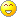epsilonSenior MemberJoined: 30/07/2020
Location: Belgium
Posts: 170
 Posted: 07:58pm 12 Apr 2021Copy link to clipboardPrint this post

matherp said  The example is correct. map(134) is used to output the text in the colour rgb(149,73,66) which has been loaded into clut slot 134 which is found by map(134) returning &H802080 which the firmware converts to &B10000110 which = 134

You're right of course. I should have thought that through before responding. This is almost identical to the discussion about the color map in the Mapster thread.
The caveat being that despite the assignment on line 1, MAP(134) <> RGB(149,74,66), but the example never claimed it would be so.
Epsilon CMM2 projects

frnno967
Regular Member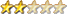Joined: 02/10/2020
Location: United States
Posts: 81
 Posted: 01:23am 13 Apr 2021Copy link to clipboardPrint this post

Peter, I believe the new GUI CURSOR capability may have a bug. When the coordinates are being updated rapidly the sprite flickers. In maxiterm I'm using the GUI CURSOR SHOW and HIDE commands to show an "underline" sprite and flash it on and off. In the main loop of the program the coordinates are updated rapidly every cycle as the loop runs. The show and hide are operating correctly but there's a noticeable flicker in the sprite as it's coordinates are updated.

You can see a video of it in action via this link.

It's not just a refresh issue with my monitor that I can tell, and only started flickering after I changed the code to take advantage of the SHOW and HIDE functionality of the latest beta firmware (b26).

If you want to test it for yourself, just run maxiterm and hit ALT-U after the initial prompts:
maxiterm 1.9.5 revised.zip

Thank you.
Edited 2021-04-13 11:32 by frnno967
Ham Radio Operator, K5JCJ. Computer Enthusiast. Musician. Engineer.

matherp
GuruJoined: 11/12/2012
Location: United Kingdom
Posts: 4738
 Posted: 07:05am 13 Apr 2021Copy link to clipboardPrint this post

Quote  When the coordinates are being updated rapidly the sprite flickers.

Of course, the update hides and then re-shows so if you sit in a tight loop it will flicker. Use any of the normal graphic techniques (page copy, getscanline etc.) to mitigate this or just slow down the update rate
Edited 2021-04-13 17:10 by matherpPage 5 of 5Print this page

To reply to this topic, you need to log in.

© JAQ Software 2021